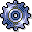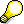Transaction-Based Material Price DeterminationUse

This function makes it possible for you to calculate the moving average price after every goods movement.

• If the material ledger is not active, you can only calculate the moving average price in the company code currency.
• If the Material Ledger is active, you can calculate the moving average prices for materials in up to three currencies/valuations.

Integration

After each valuation-relevant transaction, the system automatically performs price determination to adjust the valuation price of the material and the value of the material stock.

Prerequisites

If you want to calculate the moving average price in up to three currencies/valuations, check the following settings:

• The material ledger is active for the material.
• The material has material price determination 2.
• The material has price control indicator V.If the material has material price determination 2 and price control S, the moving average price is calculated for information only. The material is valuated with the standard price.

Features

Transaction-based material price determination takes the following amounts into account:

• Price differences
• Exchange rate differences
• Revaluation amounts

The standard price can be calculated statistically for materials having price control indicator V, but it has no impact on the valuation of the material.

Postings for Transaction-Based Material Price Determination

The posting logic of transaction-based price determination corresponds to that of the system without the material ledger. Two accounting documents and a Material Ledger document are created for transaction-based material price determination. One of the accounting documents documents the updating of costs in accordance with the expected material price. The other accounting document documents the distribution of price and exchange rate differences. The Material Ledger document exists as a follow-on document to the material document.